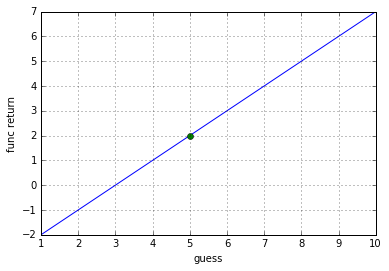# Equation Solving¶

ultimately want to discover n roots programatically

In :
%matplotlib inline
import numpy as np
import matplotlib.pyplot as plt
from scipy.optimize import fsolve


## Finding a Single Root¶

In this small equation, x should correctly equal 5.

f(x) is a coded representation of the written problem.
f(x) takes a possible numeric solution as its only argument.
if f(x) ever returns 2, then the guess is correct.

$$-3 + x = 2$$
In :
f = lambda x: -3 + x


### Brute Force Approach¶

First, I will solve for x with brute force guessing by passing various guesses into f(x) in form of array.

In :
xGuesses = np.array([1,2,3,4,5,6,7,8,9,10])

print("func results:", f(xGuesses))

func results: [-2 -1  0  1  2  3  4  5  6  7]


Array output may shows the sequence of answers with respect to the input order
this will become the y position on the plot

In :
plt.plot(xGuesses, f(xGuesses))
plt.plot(5, 2, 'o')
plt.xlabel("guess")
plt.ylabel("func return")
plt.grid()
plt.show()The grid shows that 5 intersects with 2. Since our desired output is 2, 5 is my answer.

Another way to discover a solution is to use scipy numerical solver to find the roots

Instead of visually hunting for the answer in a grid, one can simply be given a solution in plain text.

As far as I know, scipy.fsolve thinks that when a function returns "zero", then that is the correct solution Therefore, I must modify the equation to equal zero instead of 2.

$$-3 + x -2 = 0$$
In :
f = lambda x: -3 + x -2

In :
initial_guess = 1
solution = fsolve(f, initial_guess)

print ("The solution is x = %f" % solution)

The solution is x = 5.000000


This is very similar to the homebrew brute force, except the scipy lib has some optimizations that are helpful.

## Finding Two Roots¶

im not very good at this yet

$$2y−3x =−27$$$$5y+3x=6$$

### Brute Force Matrix¶

so I was unable to figure out how to graph this problem in an intelligible way. I did figure out how to create a matrix with all possible combinations which allowed me to use the same f(numpy.array) strategy. I thought that the matrix should be called a permutation matrix, however, there already seems to be some advanced terminology that uses the same word for a different meaning. I recall it saying "permute this matrix". I am still unsure what this means.

I am going to convert the equation to equal zero, so that i can use uniform solutions across differing equations

$$A = 2y−3x+27 = 0$$$$B = 5y+3x-6 = 0$$
In :
f = lambda x, y: (2*y) - (3*x) + 27
g = lambda x, y: (5*y) + (3*x) - 6

In :
possibleRange = list(range(-5, 10))

# n^r (permutations)
guessMatrix = np.array([np.repeat(possibleRange, len(possibleRange)),
np.tile(possibleRange, len(possibleRange))])

matches = [i for i, x in enumerate(f(*matrix).tolist()) if x == 0]
for i in matches:

print(A); print(B)
print(set(A).intersection(B))

['[7, -3]', '[9, 0]']
['[-3, 3]', '[2, 0]', '[7, -3]']
{'[7, -3]'}


python's set class object is very useful for finding differences in collections. I had to serialize the return results so that the set.intersection comparison could be made. Fortunately for me, the set.intersection also returns all points, unlike the list.index

### numpy meshgrid¶

A matrix solution I found that did not work as expected was:

guessMatrix = np.array(np.meshgrid([1, 2, 3], [4, 5], [6, 7])).T.reshape(-1,3))

[1, 4, 6]
[1, 5, 6]
[2, 4, 6]
[2, 5, 6]
[3, 4, 6]
[3, 5, 6]
[1, 4, 7]
[1, 5, 7]
[2, 4, 7]
[2, 5, 7]
[3, 4, 7]
[3, 5, 7]

The np.array(np.meshgrid([-5...10], [-5...10])).T.reshape(-1,2)) created the correct number of matches as:

[1, 4]
[1, 5]
[2, 4]
[2, 5]
[3, 4]
[3, 5]

but since I needed the array to be in pivoted form of:

[1,1,2,2,3,3]
[4,5,4,5,4,5]

I attempted to pivot the array using reshape(2,-1) with unexpected results:

[1, 4, 1, 5, 2, 4]
[2, 5, 3, 4, 3, 5]

The was expecting a perfect pivot:

[1, 1, 2, 2, 3, 3]
[4, 5, 4, 5, 4, 5]

Even though there may be a way to use these methods to achieve what i am looking for, ultimately it became unreadable and i lost my way.

Brent suggested looking up matrix transformations

In [ ]: